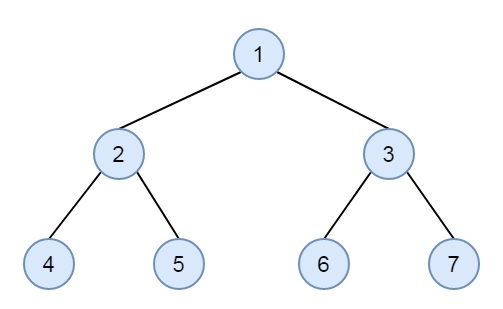# Check if a binary tree is sorted level-wise or not in C++

Here we will see how to check a binary tree is level wise sorted or not. The level wise sorted binary tree will be look like below −In each level, the nodes are sorted from left to right, and each layer contains higher value than its previous level.

We can solve this problem by performing level order traversal, and keep track of the minimum and maximum elements of current level. Use another variable prev_max to hold maximum value of the previous level. Then compare the minimum value of current level and maximum value of previous level prev_max. If min value is greater than prev_max, then the tree is sorted level wise up to current level. Then update prev_max and update the max value of current level. Repeat this until all levels are not traversed.

## Example

#include <iostream>
#include <queue>
using namespace std;
class Node {
public:
int key;
Node *left, *right;
};
Node* getNode(int key) {
Node* newNode = new Node;
newNode->key = key;
newNode->left = newNode->right = NULL;
return newNode;
}
bool isLevelWiseSorted(Node* root) {
int prevMax = INT_MIN;
int min_val, max_val;
int levelSize;
queue<Node*> q;
q.push(root);
while (!q.empty()) {
levelSize = q.size();
min_val = INT_MAX;
max_val = INT_MIN;
while (levelSize > 0) {
root = q.front();
q.pop();
levelSize--;
min_val = min(min_val, root->key);
max_val = max(max_val, root->key);
if (root->left)
q.push(root->left);
if (root->right)
q.push(root->right);
}
if (min_val <= prevMax)
return false;
prevMax = max_val;
}
return true;
}
int main() {
Node* root = getNode(1);
root->left = getNode(2);
root->right = getNode(3);
root->left->left = getNode(4);
root->left->right = getNode(5);
root->right->left = getNode(6);
root->right->right = getNode(7);
if (isLevelWiseSorted(root))
cout << "Tree is levelwise Sorted";
else
cout << "Tree is Not levelwise sorted";
}

## Output

Tree is level wise Sorted